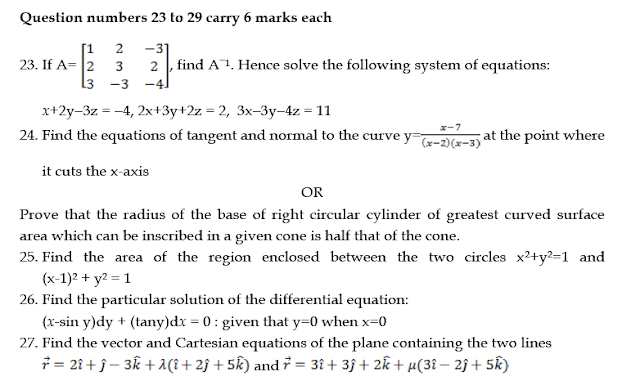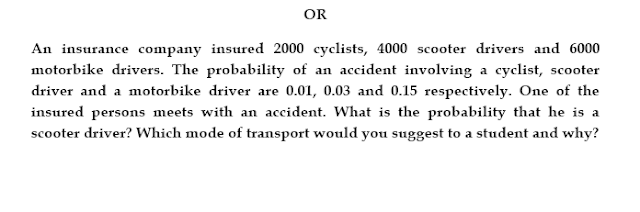# SCC Education

## SAMPLE QUESTION PAPER MATHEMATICS CLASS 12

General Instructions
1. All questions are compulsory.
2. The question paper consist of 29 questions divided into three sections A, B and C.Section A comprises of 10 questions of one mark each, section B comprises of 12 questions of four marks each and section C comprises of 07 questions of six marks each.
3. All questions in Section A are to be answered in one word, one sentence or a s per the exact requirement of the question.
4. There is no overall choice. However, internal choice has been provided in 04 questions of four marks each and 02 questions of six marks each. You have to attempt only one of the alternatives in all such quest ions.
5. Use of calculators in not permitted. You may ask for logarithmic tables, if required.To get solution click here ................................click here

solving-models-related-to-ordinary differential equation

differentiation-of-exponential-functions

area-between-curve

vector

application-of-derivatives

homogenous-differential-equation

inverse-trigonometry-questions

integration

integration-questions

Rolles-theorem-and-mean-value-theorem

Techniques-of-integration-substitution

critical-points-and-point-of-inflection

intermediate-value-theorem

### Sample paper Mathematics 12